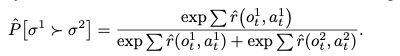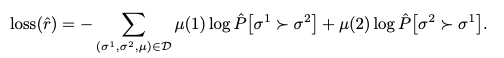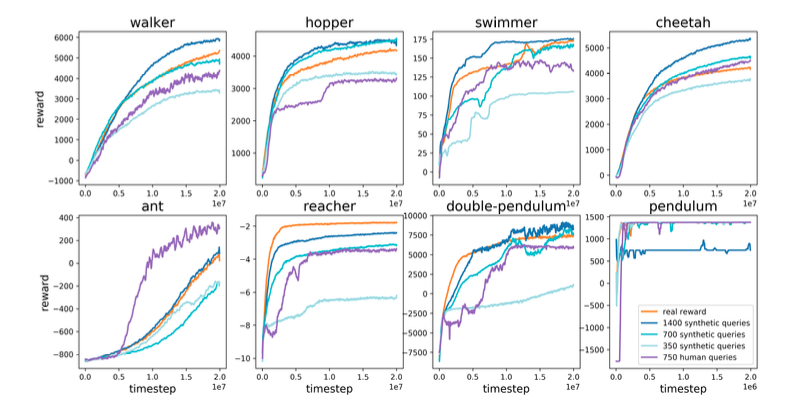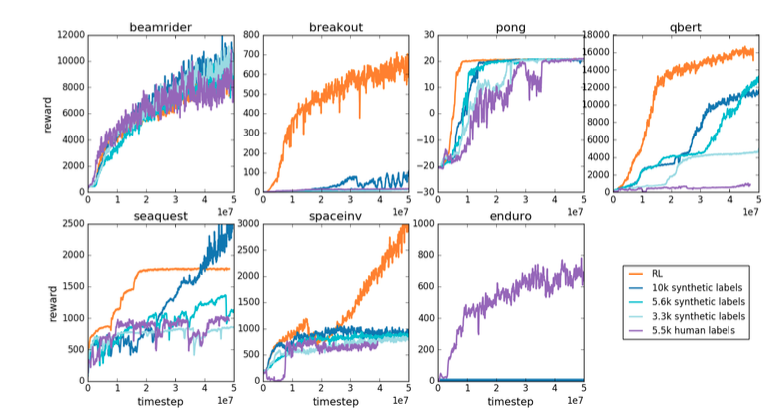# Catch up on the latest AI articles# RLHF: How To Train Reinforcement Learning Agents Using Human Evaluation

3 main points
✔️ Compare and evaluate agent behavior with human evaluations to quantify goodness and train a reward model to approximate it
✔️ Train reinforcement learning agents using the reward model
✔️ Agents trained using the reward model have shown performance comparable to agents trained using the normal performance comparable to that of agents trained using the normal reward

Deep reinforcement learning from human preferences
written by Paul ChristianoJan LeikeTom B. BrownMiljan MarticShane LeggDario Amodei
(Submitted on 12 Jun 2017 (v1), last revised 17 Feb 2023 (this version, v4))

Subjects: Machine Learning (stat.ML); Artificial Intelligence (cs.AI); Human-Computer Interaction (cs.HC); Machine Learning (cs.LG)code：The images used in this article are from the paper, the introductory slides, or were created based on them.

## Introduction

The success of reinforcement learning depends largely on the design of a good reward function, and for many tasks, the design of such a function itself is said to be difficult.

For example, if we want a robot to learn to clean a desk by reinforcement learning, we do not know what kind of reward function is appropriate, and even if we design a simple reward function, it may induce unintended behavior. Thus, the misalignment between our human values and the objective function for the reinforcement learning system has been considered a drawback in the field of reinforcement learning.

If ideal demonstration data for a task are available, the behavior can be copied by inverse reinforcement learning or imitation learning, but in situations where such data are not available, these methods are not applicable.

An alternative approach was to have humans evaluate the system's behavior and provide feedback. However, using human feedback as a reward is not realistic, considering that reinforcement learning requires a large amount of feedback from experience.

To overcome these challenges, this paper employs a method of learning a reward function using feedback data from human evaluations, which is then used to optimize the reinforcement learning system. Although such a method has been proposed in older research, this paper applies it to the recent framework of deep reinforcement learning to obtain more complex behaviors.

## Technique

Assuming $\pi$ as the strategy and $\hat{r}$ as the estimated reward function, the following three processes are repeated to train the RL agent.

First, in process 1, the strategy $\pi$ is used to collect time-series data (trajectories)$\tau$ of interactions with the environment. The strategy is optimized using a conventional reinforcement learning algorithm to maximize the cumulative sum of $\hat{r}$.

Next, in Process 2, we select two short segments $\sigma$ from the set of time series data $\tau$ obtained in Process 1, and ask a human to compare and evaluate which is better.

In Process 3, we will use the human evaluation feedback to optimize the estimated reward function $\hat{r}$.

### Policy optimization

The estimated reward function $\hat{r}$ can be used with the usual reinforcement learning algorithms, but one thing to keep in mind is that $\hat{r}$ is not static. Therefore, policy gradient methods that are robust to changes in the reward function should be employed, which is why A2C [Mnih et al., 2016] and TRPO [Schulman et al., 2015] are used in this study.

The reward values generated by $\hat{r}$ are normalized to have a fixed standard deviation and mean of 0.

### Preferred Use

Evaluators are presented with two trajectory segments $\sigma$ in the form of a short movie of 1~2 seconds and rate them by comparing which they prefer. Human ratings are expressed as a distribution $\mu$.

### Learning Reward Functions

The loss function is calculated as follows## Experimental results

Evaluators are presented with a short video of a trajectory segment of one or two seconds and are asked to rate which segment is better, repeating the process hundreds to thousands of times. Thus, each person spends from 30 minutes to 5 hours providing feedback.

### Robot simulation

The first task is a series of eight robot-based tasks (walker, hopper, swimmer, cheetah, ant, reacher, double-pendulum, pendulum) implemented on a physics simulator called MuJoCo.The result is shown above.

When the reward model is trained on human evaluation data and the agent is trained using the output of the model as the reward (purple) and

When the reward model is trained with the real reward values of the environment and the agent is trained using the output of the model as the reward (blue system) and

The performance of the agent on various tasks is compared with that of an agent trained using the real reward values as they are (orange).

We can see that even when using the proposed reward model (purple and blue), the results are comparable to those obtained when training agents using real reward values (orange), as in the case of normal reinforcement learning.

### Atari

The second task is a task using Atari's seven games (beamrider, breakout, pong, qbert, seaquest, spaceinv, and enduro).The result is as shown above.

Depending on the type of game, we can see that the proposed method using the reward model (purple and blue series) wins or loses to the regular reinforcement learning method (orange).

For most games, training the reward model using human feedback (purple) performs as well or slightly worse than training the reward model with real reward values (blue series), but given the number of data used to train the reward model However, considering the number of data used to train the reward model, the former (purple) seems to be more efficient.

### Supplemental experimental results

The proposed method of having the reward model learn human evaluations is expected to be particularly effective in tasks where it is difficult to set up a good reward function.

The above robot simulation experiments, in which the hopper robot learned to do somersaults and the half-cheetah robot learned to move forward on one leg, were also successful in learning behaviors that would normally require complex reward function settings in a short period of time.

The ablation study also revealed the following points

First, we found that training the reward model offline, i.e., using the accumulated behavior data instead of the most recent agent's behavior, resulted in the acquisition of undesirable behavior. This indicates that feedback on human evaluations needs to be provided online.

We also asked the human raters to feed back their ratings by comparing two examples, rather than absolute scores, in order to obtain consistent ratings, which was especially true in the continuous control task. The reason for this may be that when the reward scale changes significantly, regression methods that predict absolute scores have difficulty predicting rewards.

## Conclusion

We have shown that the method of training reward prediction reward models using feedback from human evaluations is also effective in recent deep reinforcement learning systems. This is an important first step toward applying deep reinforcement learning to complex real-world tasks.

## Summary

In this article, we introduced a recent paper on Reinforcement learning from Human Feedback (RLHF), a well-known method for fine-tuning large-scale language models to exhibit behavior consistent with human values. Since the publication of this paper in 2017, many methods have been proposed for RLHF, and it is an important field that is expected to continue to develop. We hope this article will help you keep up with RLHF trends.

If you have any suggestions for improvement of the content of the article,